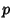## Sylow Theorems

Letbe a Prime Number,a Group, andthe order of.

1. Ifdivides, thenhas a Sylow p-Subgroup.

2. In a Finite Group, all the Sylow p-Subgroup are isomorphic for some fixed.

3. The number of Sylow p-Subgroup for a fixedis Congruent to 1 (mod).